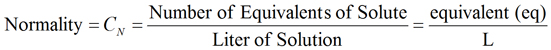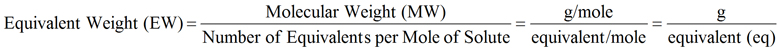PhysiologyWeb  Home  |  FAQ  |  About  |  Contact  |   - Sign InTweetNormal Solution Concentration Calculator
In some cases, particularly in situations involving acid-base chemistry, the solution concentration is expressed in normality (N or CN). Normality is defined as the number of equivalent weights (or simply equivalents, eq) of solute dissolved per liter of solution (equivalents/L = N) (Equation 1). Normality is used in place of molarity because often 1 mole of acid does not neutralize 1 mole of base. Thus, in order to have a one-to-one relationship between acids and bases, many chemists prefer to express the concentration of acids and bases in normality. Similar to molarity, where the molecular weight (MW) is used for calculating the molar concentration, for normal solution concentrations, it is desirable to use the equivalent weight (EW). For acids, the equivalent weight (EW) is defined as the mass (in grams) of an acid that provides exactly 1 mole of hydrogen ions (H+) (to be precise, hydronium ions, H3O+) to a reaction. Another way of expressing this is that the equivalent weight is defined as the mass (in grams) of an acid that reacts with exactly 1 mole of hydroxide ions (OH). For bases, the equivalent weight is defined as the mass (in grams) of a base that provides exactly 1 mole of hydroxide ions (OH) to a reaction. Another way of expressing this is that the equivalent weight is defined as the mass (in grams) of a base that reacts with exactly 1 mole of hydrogen ions (H+). Because 1 mole of acid or base is equal to 1 equivalent of acid or base, equivalent weight is expressed in grams per equivalent (g/equivalent or g/eq) (Equation 2). While it is more appropriate to refer to equivalent weight as equivalent mass, this term is rarely used.(Equation 1)

As stated above, the equivalent weight (EW, expressed in grams per equivalent or g/eq) is related to the chemical nature of the acid or base under consideration. It is calculated by dividing the molecular weight (MW) of an acid or base by the number of equivalents per mole for that acid or base (Equation 2). For acids, the number of equivalents per mole is the number of moles of hydrogen ions (H+) contributed to the reaction per mole of acid. For bases, the number of equivalents per mole is the number of hydroxide ions (OH) contributed to the reaction per mole of base. Thus, knowledge of the chemical nature of the acid and/or base involved in the chemical reaction is necessary to determine the equivalent weight of the acid or base. Tables 1 and 2 provide a few examples of acids (Table 1) and bases (Table 2), the number of equivalents per mole for each acid or base, and the corresponding equivalent weight values.(Equation 2)

Table 1. A few examples of acids and their values for molecular weight, number of equivalents per mole of acid, and equivalent weight
 Acid Molecular Weight(g/mol) Number of Equivalents per Mole of Acid Equivalent Weight(g/equivalent = g/eq) Hydrochloric acid (HCl) 36.46 g/mol 1 36.46 g/eq Hydrobromic acid (HBr) 80.91 g/mol 1 80.91 g/eq Hydroiodic acid (HI) 127.91 g/mol 1 127.91 g/eq Chloric acid (HClO3) 84.46 g/mol 1 84.46 g/eq Perchloric acid (HClO4) 100.46 g/mol 1 100.46 g/eq Nitric acid (HNO3) 63.01 g/mol 1 63.01 g/eq Sulfuric acid (H2SO4) 98.08 g/mol 2 49.04 g/eq
Note: For an acid, the number of equivalents per mole of acid is the number of moles of hydrogen ion (H+) provided by 1 mole of acid in a reaction, or the number of moles of hydroxide ions (OH) neutralized by 1 mole of acid in a reaction.

Table 2. A few examples of bases and their values for molecular weight, number of equivalents per mole of base, and equivalent weight
 Base Molecular Weight(g/mol) Number of Equivalents per Mole of Base Equivalent Weight(g/equivalent = g/eq) Lithium hydroxide (LiOH) 23.95 g/mol 1 23.95 g/eq Sodium hydroxide (NaOH) 40.00 g/mol 1 40.00 g/eq Potassium hydroxide (KOH) 56.11 g/mol 1 56.11 g/eq Calcium hydroxide, Ca(OH)2 74.09 g/mol 2 37.05 g/eq Barium hydroxide, Ba(OH)2 171.34 g/mol 2 85.67 g/eq
Note: For a base, the number of equivalents per mole of base is the number of moles of hydroxide ions (OH) provided by 1 mole of base in a reaction, or the number of moles of hydrogen ions (H+) neutralized by 1 mole of base in a reaction.

Given the above information, the normal concentration of a solution can be calculated by using Equation 3, where CN is the normality or normal concentration, m is the mass of acid or base used, V is the total solution volume, and EW is the equivalent weight.(Equation 3)

The normal concentration of a solution (normality, CN) is always equal to or greater than the molar concentration (molarity, CM) of the solution. The normal concentration can be calculated by multiplying the molar concentration by the number of equivalents per mole of solute (Equation 4). See Tables 1 and 2 for some typical values for the number of equivalents per mole of acid (Table 1) or base (Table 2).(Equation 4)

It should also be noted that normality and equivalents are not used only in acid-base chemistry, but also in other applications such as reduction-oxidation (redox) reactions. Finally, it should be noted that the concept of normality evolved before the concept of molarity. Today, normality is not as widely used as it was by chemists a generation ago.
Meant to be used in both the teaching and research laboratory, this calculator (see below) can be utilized to perform a number of different calculations for preparing normal solutions when starting with the solid material. For example, the equivalent weight of a chemical can be used along with the desired solution volume and solute concentration to determine the mass of chemical needed to make such a solution. On the other hand, if the desired concentration is known, but only a small amount (i.e., mass) of the chemical is available (such as when a very small amount is purchased, e.g., 10 mg), then the total solution volume can be calculated in which the solid material must be dissolved to achieve the desired final concentration.
If you wish to perform dilution calculations for solutions with normality concentration units, use our Normality Dilution Calculator. If you are starting with the solid material and wish to make a solution with the concentration expressed in molarity, use our Molar Solution Concentration Calculator.
If you wish to perform dilution factor or fold dilution calculations for solutions with normality concentration units, use our Dilution Factor Calculator - Normality.
Normal solution concentration equation• CN is the normal concentration in equivalents/L (Normal or N). This is also referred to as normality, which is used to express the concentration of a solute in a solution. Normality is defined as the number of equivalents of solute dissolved per liter of solution (equivalents/L = N) (Equations 1, 3, and 4). A 1 N solution is one in which exactly 1 equivalent of solute is dissolved in a total solution volume of exactly 1 L. The normal concentration (CN) is always equal to or greater than the molar concentration (CM) (Equation 3). Using SI prefixes, the concentration may also be expressed in different fractions of the normal concentration such as milliequivalents/L (mN), microequivalents/L (μN), nanoequivalents/L (nN), picoequivalents/L (pN), etc.
• m is the mass (i.e., weight) of solute in grams (g) that must be dissolved in volume V of solution to make the desired normal concentration (CN).
• V is volume of solution in liters (L) in which the indicated mass (m) of solute must be dissolved to make the desired normal concentration (CN). Note that V is the final or total volume of solution after the solute has been added to the solvent.
• EW is the equivalent weight in g/equivalent. It is calculated by dividing the molecular weight of solute by the number of equivalents per mole of solute (Equation 2). For acids, the number of equivalents per mole is the number of H+ ions contributed by the acid per mole of acid. For bases, the number of equivalents per mole is the number of OH ions contributed by the base per mole of base. The molecular weight of an acid or base can be obtained from the molecular formula, data tables, or the label on the bottle containing the acid or base of interest.
Normal solution concentration calculator
Each calculator cell shown below corresponds to a term in the formula presented above. Enter appropriate values in all cells except the one you wish to calculate. Therefore, at least three cells must have values, and no more than one cell may be blank. The value of the blank cell will be calculated based on the other values entered. After a calculation is performed, the calculated cell will be highlighted and subsequent calculations will calculate the value of the highlighted cell (with no requirement to have a blank cell). However, a blank cell has priority over a highlighted cell.
For convenience, this worksheet allows you to select different mass, volume, and concentration units, and the necessary conversions are carried out for you to obtain the value of the blank cell in the desired unit. Note that the unit of equivalent weight must be g/equivalent.
 Calculate Equivalent Weight Molecular weight g/mol Number of equivalents

 Equivalent weight g/equivalent Mass of solute g pg ng μg mg g kg Total solution volume mL fL pL nL μL mL L Concentration mN fN pN nN μN mN N